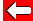Solving equations like ax² + c = 0 can be quite straightforward.
e.g. x² - 25 = 0

From your work on algebra, you should be able to rearrange the equation to: x² = 25

By taking the square-root of both sides, we end up with:
x = 5

That wasn't too bad, was it? Another solution is x = -5, but we'll look at that another time.

Here's one for you. Find the solution to the equation: x² - 121 = 0.Go back a pageMaths Menu